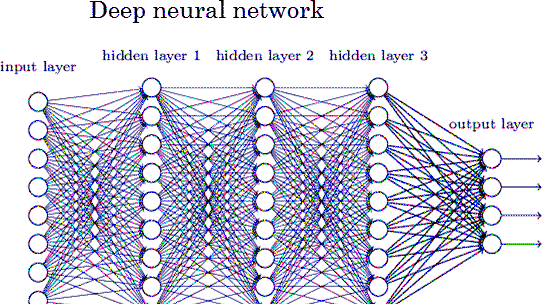# Handwritten digit recognition (deep learning,tutorial,very simple)

By crypt0z | crypto0z | 2 Feb 2021

## - Introduction

in this post, we will build our Handwritten digit recognition neural network, which is the "hello, world" programme in deep learning
you don't need to deeply understand how neural network works, but you can think of them as a mathematical model that tries to make sense of numbers
neural networks consist of input layers, hidden layers and an output layer that holds the probability of that image belongs to this category

I recommend you to watch this video which explains how neural networks work

here is an image to clarify what i mean :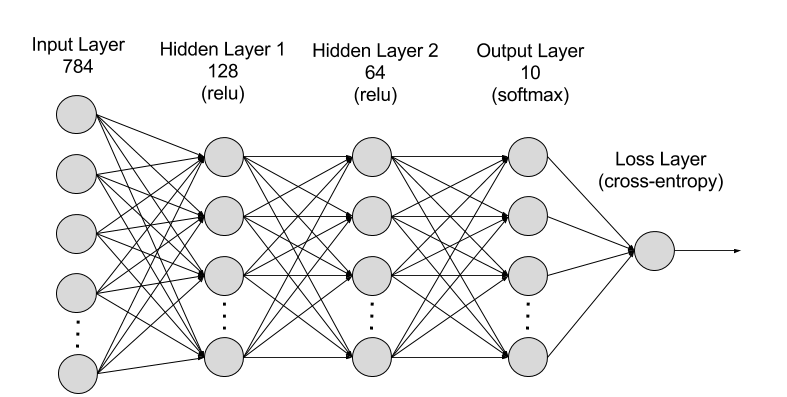## - Requirements

###### pip install tensorflow

pip install keras

## - Preparing Data

we will start our programme by importing the dataset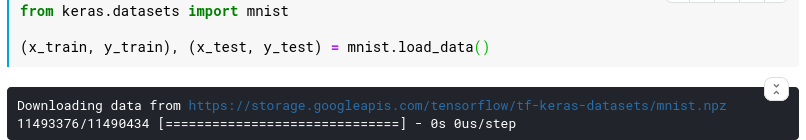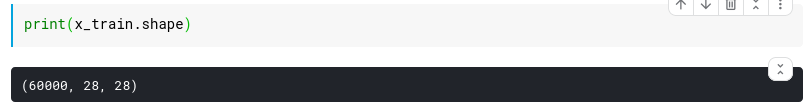after loading the dataset, we need to make it in a way that our model can understand, as neural networks only understand numbers

Reshaping : we need to reshape the data because, in deep learning, we provide the intensities of the raw pixels to our neural nets, so here our model will expect an image that has a shape of (784,), which is the flattened version of the image (28*28=784)

to reshape the images write this code :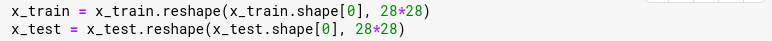Data type: after reshaping, we need to change the pixel intensities to float32 datatype so that we have a uniform representation throughout the solution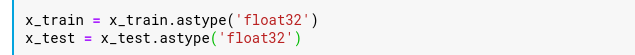Normalize : we here normalize these floating points values in the range [0-1] to improve computational efficiency as well as to help the model to generalize better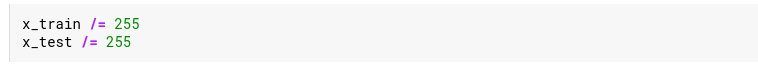One Hot Encoding: the class labels for our neural network to predict are numeric digits ranging from [0-9], as it's a multi-label classification which means the output layer in the neural network will calculate the probability of this image belongs to this category, for example, our model output might be something like this : [0.1,0.1,0.1,0.1,0.1,0.1,0.1,0.1, 0.15, 0.05], in this case, we will take the highest probability in the array which will be index 8, and the final output will be like this : [0, 0, 0, 0, 0, 0, 0, 0, 1, 0] and this called one-hot encoding, now we need to turn our labels to be like the previous array

to do that, write this code :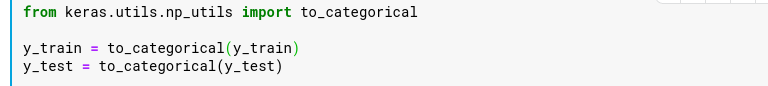## - Model

i

in this post, we will use a simple neural network with 784 input neurons (the shape of our reshaped images), and we will use three hidden layers:

hidden-layer-1 : 128 neurons with "Relu" activation function

hidden-layer-2 : 256 neurons with "Relu" activation function

hidden-layer-3 : 512 neurons with "Relu" activation function

output-layer: in this layer, we will have the number of neurons to be 10, as each neuron correspond to different class and holds it's probability, we will use "softmax" activation function because this a multi-classification problem

here is the code :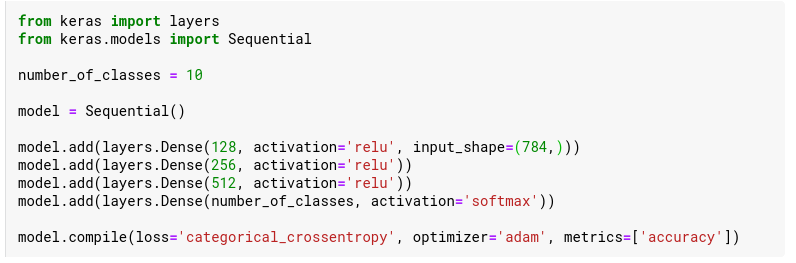in the last line, we told the model how to learn and what to focus on during learning, which is "accuracy", we used "categorical_crossentropy" as a loss function because this multi-classification problem, we also used our optimizer to be "adam" (gradient descent algorithm)

now we need to 'train' our model, here is the code :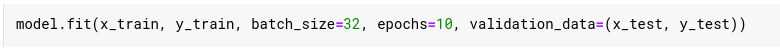let's explain what that line means, first we provided the fit() method with (x_train, y_train) which our dataset that the model will learn from, then batch_size means that after processing 32 images update the "weights" of the neurons. epochs mean how much the model will iterate through the whole dataset, in our case, it's 10 times. "validation_data" is just evaluation of our model using a different dataset, (x_test, y_test), that the model has never seen before

here is the output of the previous line :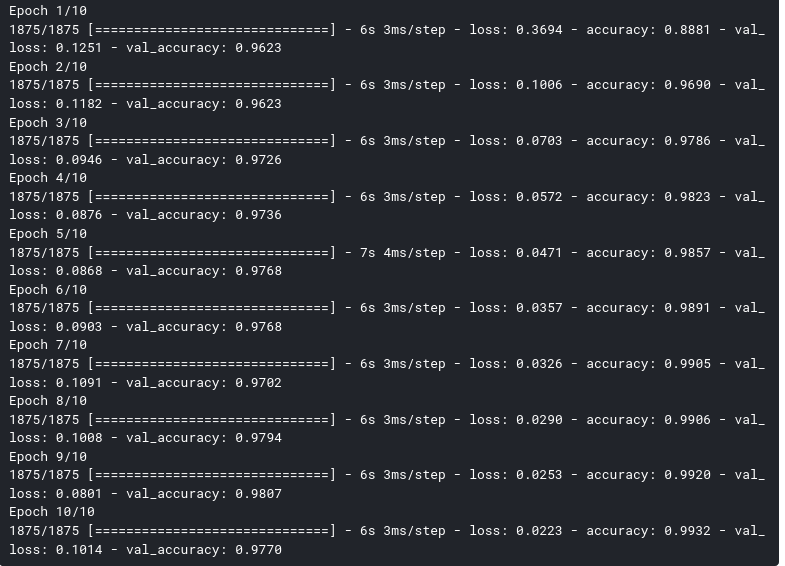let's evaluate our model :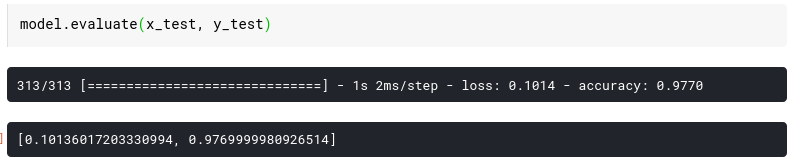we got 97% accuracy with our model, which is pretty cool

of course, you can make the model achieve better accuracy with tuning the number of hidden layers and neurons or adding "Dropout" or use  CNN,  but because this our first model in deep learning i wanted to make it simple to follow.

hope that was helpful : )crypto0z

hello <3Send a \$0.01 microtip in crypto to the author, and earn yourself as you read!

20% to author / 80% to me.
We pay the tips from our rewards pool.